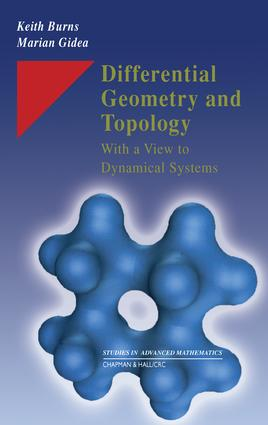# Differential Geometry and Topology

## With a View to Dynamical Systems, 1st Edition

Chapman and Hall/CRC

400 pages | 132 B/W Illus.

##### Purchasing Options:\$ = USD
Hardback: 9781584882534
pub: 2005-05-27
SAVE ~\$28.00
\$140.00
\$112.00
x
eBook (VitalSource) : 9780429124006
pub: 2005-05-27
from \$70.00

FREE Standard Shipping!

### Description

Accessible, concise, and self-contained, this book offers an outstanding introduction to three related subjects: differential geometry, differential topology, and dynamical systems. Topics of special interest addressed in the book include Brouwer's fixed point theorem, Morse Theory, and the geodesic flow.

Smooth manifolds, Riemannian metrics, affine connections, the curvature tensor, differential forms, and integration on manifolds provide the foundation for many applications in dynamical systems and mechanics. The authors also discuss the Gauss-Bonnet theorem and its implications in non-Euclidean geometry models.

The differential topology aspect of the book centers on classical, transversality theory, Sard's theorem, intersection theory, and fixed-point theorems. The construction of the de Rham cohomology builds further arguments for the strong connection between the differential structure and the topological structure. It also furnishes some of the tools necessary for a complete understanding of the Morse theory. These discussions are followed by an introduction to the theory of hyperbolic systems, with emphasis on the quintessential role of the geodesic flow.

The integration of geometric theory, topological theory, and concrete applications to dynamical systems set this book apart. With clean, clear prose and effective examples, the authors' intuitive approach creates a treatment that is comprehensible to relative beginners, yet rigorous enough for those with more background and experience in the field.

### Reviews

"The authors introduce important concepts by means of intuitive discussions and suggestive examples and follow them with significant applications, especially those related to dynamics. …The authors have succeeded in the integration of geometric theory, topological theory, and concrete applications to dynamical systems."

-Mathematical Reviews, Andrew Bucki

"The authors of this book treat a great many topics very concisely."

-MAA Reviews, William J. Satzer, 2005

"A noteworthy feature of the presentation is that dynamical systems, which are introduced in the second chapter, are used systematically to illustrate concepts and as a source of applications."

-CMS Notes, Vol. 38, No. 2, March, 2006

". . . very well written, in a very pedagogical manner and it covers a lot of material in a very clear way. I think this is an ideal introduction to differential geometry and topology for beginning graduate students or advanced undergraduate students in mathematics, but it will be, also, useful to physicist or other scientists with an interest in differential geometry and dynamical systems."

– Paul Blaga, in Babes- Bolyai Mathematica, June 2007, Vol. 52, No. 2

"Numerous illustrations and exercises round off the picture of an original and very readable textbook."

– M. Kunzinger, in Monatshefte fur Math, 2007, Vol. 152, No. 1

### Table of Contents

MANIFOLDS

Introduction

Review of topological concepts

Smooth manifolds

Smooth maps

Tangent vectors and the tangent bundle

Tangent vectors as derivations

The derivative of a smooth map

Orientation

Immersions, embeddings and submersions

Regular and critical points and values

Manifolds with boundary

Sard's theorem

Transversality

Stability

Exercises

VECTOR FIELDS AND DYNAMICAL SYSTEMS

Introduction

Vector fields

Smooth dynamical systems

Lie derivative, Lie bracket

Discrete dynamical systems

Hyperbolic fixed points and periodic orbits

Exercises

RIEMANNIAN METRICS

Introduction

Riemannian metrics

Standard geometries on surfaces

Exercises

RIEMANNIAN CONNECTIONS AND GEODESICS

Introduction

Affine connections

Riemannian connections

Geodesics

The exponential map

Minimizing properties of geodesics

The Riemannian distance

Exercises

CURVATURE

Introduction

The curvature tensor

The second fundamental form

Sectional and Ricci curvatures

Jacobi fields

Manifolds of constant curvature

Conjugate points

Horizontal and vertical sub-bundles

The geodesic flow

Exercises

TENSORS AND DIFFERENTIAL FORMS

Introduction

Vector bundles

The tubular neighborhood theorem

Tensor bundles

Differential forms

Integration of differential forms

Stokes' theorem

De Rham cohomology

Singular homology

The de Rham theorem

Exercises

FIXED POINTS AND INTERSECTION NUMBERS

Introduction

The Brouwer degree

The oriented intersection number

The fixed point index

The Lefschetz number

The Euler characteristic

The Gauss-Bonnet theorem

Exercises

MORSE THEORY

Introduction

Nondegenerate critical points

The gradient flow

The topology of level sets

Manifolds represented as CW complexes

Morse inequalities

Exercises

HYPERBOLIC SYSTEMS

Introduction

Hyperbolic sets

Hyperbolicity criteria

Geodesic flows

Exercises

References

Index

### Subject Categories

##### BISAC Subject Codes/Headings:
MAT000000
MATHEMATICS / General
MAT012000
MATHEMATICS / Geometry / General
MAT022000
MATHEMATICS / Number Theory
SCI040000
SCIENCE / Mathematical Physics